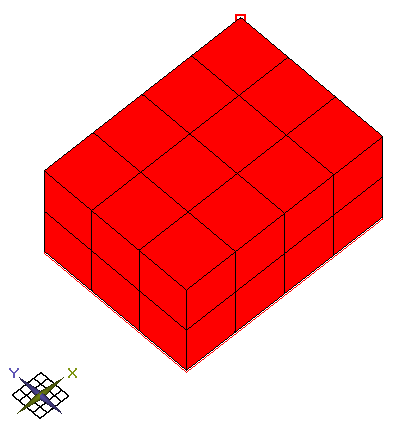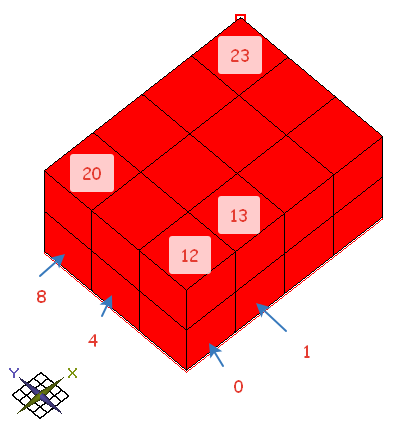Posted on

# Combine blocks

github

There is a block modelX axis – 4 blocks
Y axis – 3 blocks
Z axis – 2 blocks

Overall: 24 blocks.

Let’s number the blocksHow many the shortest paths are there between block  and ?

How long the shortest path? The length is 6 steps = 3 steps by X axis + 2 steps by Y axis and 1 step by Z axis.

The number of paths is a number of permutation with repetition = 6! / (3!*2!*1!) = 720 / (6*2*1) = 60

Another way to calc the number of paths is to create a graph and adjacency matrix.

Then we should multiply the matrix by itself 6 times and we get a number of paths of length of 6 steps between vertices.

Here we get interesting conclusion – if number of paths that are got by both two methods is equal, then the block model has all 24 blocks.

This site uses Akismet to reduce spam. Learn how your comment data is processed.